## 【Java从0到1学习】10 Java常用类汇总

article2023/9/25 14:49:02/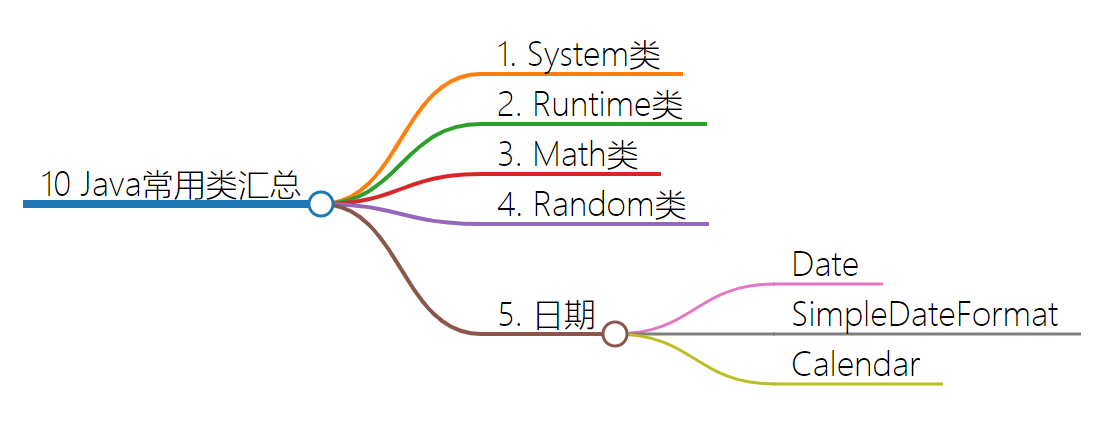## 1. System类

System类对读者来说并不陌生，因为在之前所学知识中，需要打印结果时，使用的都是“System.out.println();”语句，这句代码中就使用了System类。System类定义了一些与系统相关的属性和方法，它所提供的属性和方法都是静态的，因此，想要引用这些属性和方法，直接使用System类调用即可。System类的常用方法如下表所示。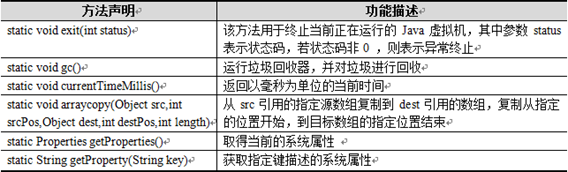1．getProperties()方法

System类的getProperties()方法用于获取当前系统的全部属性，该方法会返回一个Properties对象，其中封装了系统的所有属性，这些属性是以键值对形式存在的。

``````package cn.itcast.chapter05.example09;
import java.util.*;
/**
* System类的getProperties()方法
*/
public class Example09 {
public static void main(String[] args) {
// 获取当前系统属性
Properties properties = System.getProperties();
// 获得所有系统属性的key，返回Enumeration对象
Enumeration propertyNames = properties.propertyNames();
while (propertyNames.hasMoreElements()) {
// 获取系统属性的键key
String key = (String) propertyNames.nextElement();
// 获得当前键key对应的值value
String value = System.getProperty(key);
System.out.println(key + "--->" + value);
}
}
}

``````

2．currentTimeMillis()

currentTimeMillis()方法返回一个long类型的值，该值表示当前时间与1970年1月1日0点0分0秒之间的时间差，单位是毫秒，通常也将该值称作时间戳。

``````package cn.itcast.chapter05.example10;
/**
* 计算程序在进行求和操作时所消耗的时间
*/
public class Example10 {
public static void main(String[] args) {
long startTime = System.currentTimeMillis();// 循环开始时的当前时间
int sum = 0;
for (int i = 0; i < 100000000; i++) {
sum += i;
}
long endTime = System.currentTimeMillis();// 循环结束后的当前时间
System.out.println("程序运行的时间为：" + (endTime - startTime) + "毫秒");
}
}
``````

3．arraycopy(Object src,int srcPos,Object dest,int destPos,int length)

arraycopy()方法用于将一个数组中的元素快速拷贝到另一个数组。其中的参数具体作用如下：

• src：表示源数组。
• dest：表示目标数组。
• srcPos：表示源数组中拷贝元素的起始位置。
• destPos：表示拷贝到目标数组的起始位置。
• length：表示拷贝元素的个数。
``````package cn.itcast.chapter05.example11;
/**
* 数组元素的拷贝
*/
public class Example11 {
public static void main(String[] args) {
int[] fromArray = { 101, 102, 103, 104, 105, 106 }; // 源数组
int[] toArray = { 201, 202, 203, 204, 205, 206, 207 }; // 目标数组
System.arraycopy(fromArray, 2, toArray, 3, 4); // 拷贝数组元素
// 打印目标数组中的元素
for (int i = 0; i < toArray.length; i++) {
System.out.println(i + ": " + toArray[i]);
}
}
}
``````

4．SystemClock.uptimeMillis()

## 2. Runtime类

Runtime类用于表示虚拟机运行时的状态，它用于封装JVM虚拟机进程。每次使用java命令启动虚拟机都对应一个Runtime实例，并且只有一个实例，因此该类采用单例模式进行设计，对象不可以直接实例化。若想在程序中获得一个Runtime实例，只能通过以下方式：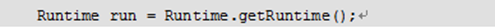``````package cn.itcast.chapter05.example12;
/**
* Runtime类的使用
*/
public class Example12 {
public static void main(String[] args) {
Runtime rt = Runtime.getRuntime(); // 获取
System.out.println("处理器的个数: " + rt.availableProcessors() + "个");
System.out.println("空闲内存数量: " + rt.freeMemory() / 1024 / 1024 + "M");
System.out.println("最大可用内存数量: " + rt.maxMemory() / 1024 / 1024 + "M");
}
}
``````

``````package cn.itcast.chapter05.example13;
import java.io.IOException;
/**
* 使用exec()方法打开记事本
*/
public class Example13 {
public static void main(String[] args) throws IOException {
Runtime rt = Runtime.getRuntime(); // 创建Runtime实例对象
}
}
``````

``````package cn.itcast.chapter05.example14;
/**
* 打开的记事本并在3秒后自动关闭
*/
public class Example14 {
public static void main(String[] args) throws Exception {
Runtime rt = Runtime.getRuntime(); // 创建一个Runtime实例对象
process.destroy(); // 杀掉进程
}
}
``````
``````Runtime.getRuntime().availableProcessors(); // 获取CPU核心数
Runtime.getRuntime().maxMemory(); // 获取应用被分配的最大内存
``````

## 3. Math类

Math类是数学操作类，Math类提供了常用的一些数学函数，如：三角函数、对数、指数等。一个数学公式如果想用代码表示，则可以将其拆分然后套用Math类下的方法即可。

Math类中有两个静态常量PI和E，分别代表数学常量π和e。

``````Math.abs(12.3);                 //12.3 返回这个数的绝对值
Math.abs(-12.3);                //12.3

Math.copySign(1.23, -12.3);     //-1.23,返回第一个参数的量值和第二个参数的符号
Math.copySign(-12.3, 1.23);     //12.3

Math.signum(x);                 //如果x大于0则返回1.0，小于0则返回-1.0，等于0则返回0
Math.signum(12.3);              //1.0
Math.signum(-12.3);             //-1.0
Math.signum(0);                 //0.0

//指数
Math.exp(x);                    //e的x次幂
Math.expm1(x);                  //e的x次幂 - 1

Math.scalb(x, y);               //x*(2的y次幂）
Math.scalb(12.3, 3);            //12.3*2³

//取整
Math.ceil(12.3);                //返回最近的且大于这个数的整数13.0
Math.ceil(-12.3);               //-12.0

Math.floor(12.3);               //返回最近的且小于这个数的整数12.0
Math.floor(-12.3);              //-13.0

//x和y平方和的二次方根
Math.hypot(x, y);               //√（x²+y²）

//返回概述的二次方根
Math.sqrt(x);                   //√(x) x的二次方根
Math.sqrt(9);                   //3.0
Math.sqrt(16);                  //4.0

//返回该数的立方根
Math.cbrt(27.0);                //3
Math.cbrt(-125.0);              //-5

//对数函数
Math.log(e);                    //1 以e为底的对数
Math.log10(100);                //10 以10为底的对数
Math.log1p(x);                  //Ln（x+ 1）

//返回较大值和较小值
Math.max(x, y);                 //返回x、y中较大的那个数
Math.min(x, y);                 //返回x、y中较小的那个数

//返回 x的y次幂
Math.pow(x, y);
Math.pow(2, 3);                 //即2³ 即返回：8

//随机返回[0,1)之间的无符号double值
Math.random();

//返回最接近这个数的整数,如果刚好居中，则取偶数
Math.rint(12.3);                //12.0
Math.rint(-12.3);               //-12.0
Math.rint(78.9);                //79.0
Math.rint(-78.9);               //-79.0
Math.rint(34.5);                //34.0
Math.rint(35.5);                //36.0

Math.round(12.3);               //与rint相似，返回值为long

//三角函数
Math.sin(α);                    //sin（α）的值
Math.cos(α);                    //cos（α）的值
Math.tan(α);                    //tan（α）的值

//求角
Math.asin(x/z);                 //返回角度值[-π/2，π/2]  arc sin（x/z）
Math.acos(y/z);                 //返回角度值[0~π]   arc cos（y/z）
Math.atan(y/x);                 //返回角度值[-π/2，π/2]
Math.atan2(y-y0, x-x0);         //同上，返回经过点（x，y）与原点的的直线和经过点（x0，y0）与原点的直线之间所成的夹角

Math.sinh(x);                   //双曲正弦函数sinh(x)=(exp(x) - exp(-x)) / 2.0;
Math.cosh(x);                   //双曲余弦函数cosh(x)=(exp(x) + exp(-x)) / 2.0;
Math.tanh(x);                   //tanh(x) = sinh(x) / cosh(x);

//角度弧度互换  360°角=2π弧度

Math.toRadians(angdeg);         //弧度转换成角度，返回：angdeg / 180d * PI

Math.PI
``````
``````package cn.itcast.chapter05.example15;
/**
* Math类中比较常见的方法
*/
public class Example15 {
public static void main(String[] args) {
System.out.println("计算绝对值的结果: " + Math.abs(-1));
System.out.println("求大于参数的最小整数: " + Math.ceil(5.6));
System.out.println("求小于参数的最大整数: " + Math.floor(-4.2));
System.out.println("对小数进行四舍五入后的结果: " + Math.round(-4.6));
System.out.println("求两个数的较大值: " + Math.max(2.1, -2.1));
System.out.println("求两个数的较小值: " + Math.min(2.1, -2.1));
System.out.println("生成一个大于等于0.0小于1.0随机值: " + Math.random());
}
}
``````

## 4. Random类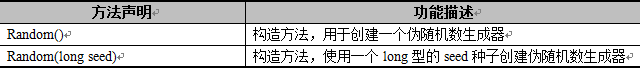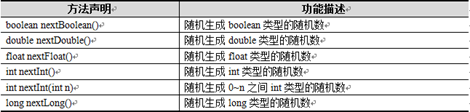``````package cn.itcast.chapter05.example16;
import java.util.Random;
/**
* 使用构造方法Random()产生随机数
*/
public class Example16 {
public static void main(String args[]) {
Random r = new Random(); // 不传入种子
// 随机产生10个[0,100)之间的整数
for (int x = 0; x < 10; x++) {
System.out.println(r.nextInt(100));
}
}
}
``````
``````package cn.itcast.chapter05.example17;
import java.util.Random;
/**
* 使用构造方法Random(long seed)产生随机数
*/
public class Example17 {
public static void main(String args[]) {
Random r = new Random(13); // 创建对象时传入种子
// 随机产生10个[0,100)之间的整数
for (int x = 0; x < 10; x++) {
System.out.println(r.nextInt(100));
}
}
}
``````
``````package cn.itcast.chapter05.example18;
import java.util.Random;
/**
* Random类中的常用方法
*/
public class Example18 {
public static void main(String[] args) {
Random r1 = new Random(); // 创建Random实例对象
System.out.println("产生float类型随机数: " + r1.nextFloat());
System.out.println("产生0~100之间int类型的随机数:" + r1.nextInt(100));
System.out.println("产生double类型的随机数:" + r1.nextDouble());
}
}
``````

## 5. 日期

getTime()
setTime()

### SimpleDateFormat

Stirngformat()格式化日期
Dateparse()

### Calendar

get(int field)返回给定日历字段的值
getInstance()
set(int year,int month,int date)设置当前日历的年月日
``````Calendar rightNow = Calendar.getInstance(); // 子类对象

public void add(int field,int amount); //根据给定的日历字段和对应的时间，来对当前的日历进行操作。

public final void set(int year,int month,int date); //设置当前日历的年月日

// 三年前的今天

``````

### 将eNSP Pro部署在华为云是什么体验

eNSP Pro简介 eNSP Pro 是华为公司数据通信产品线新推出的数通设备模拟器&#xff0c;主要应用在数据通信技能培训&#xff0c;为使用者提供华为数据通信产品设备命令行学习环境。 具备的能力 多产品模拟能力&#xff1a;支持数据通信产品线NE路由器、CE交换机、S交换机、AR…

### Redis Lua脚本执行原理和语法示例

Redis Lua脚本语法示例 文章目录 Redis Lua脚本语法示例0. 前言参考资料 1. Redis 执行Lua脚本原理1.1. 对Redis源码中嵌入Lua解释器的简要解析&#xff1a;1.2. Redis Lua 脚本缓存机制 2. Redis Lua脚本示例1.1. 场景示例1. 请求限流2. 原子性地从一个list移动元素到另一个li…